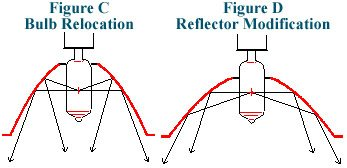# Light Analysis

Factors of Reflected Light

Measurement Of Light
Lumens is a measurement of light in all directions. This unit of measurement is best used for incandescent bulbs.

When a reflector is used, the light is reflected in one direction. Lumens is not an effective measurement of reflective light and therefore, the unit of measurement that measures light at a distance from the bulb is called candela, or more commonly referred to as foot-candles. Foot-candles can be converted to Lux by the following formula:

Foot-Candles ÷ 0.0929 = Lux

Generally speaking, foot-candles and Lux are inversely proportional to the distance squared. For example, a Lux of 200 at a distance of 5 ft. from a bulb is 50 Lux at a distance of 10 ft. In other words, if the distance is doubled the Lux is reduced to ¼ of the original Lux.

Maximizing Light Output
In order to maximize the light output using a reflector, the center of the bulb’s filament must be placed at the focal point of the reflector. The intention is to have all the light directed in a parallel manner (see Figure A). There will always be some angle of light since some light will be direct (see Figure B). A typical angle of reflection for a spot configuration is 10º.Flood Light
It is not always desirable to have a narrow light beam or a spot light. There are three ways to accomplish this, but keep in mind that light efficiency will be compromised. The first way is to position the center of the filament further from the base of the reflector’s focal point. In doing so, light is reflecting at angles other than parallel with the ends of the reflector (see Figure C). In addition, this allows for more direct light to be generated.A second method is to design the reflector at a slightly different parabolic shape so as to reflect the light at angles (see Figure D). A third way can be accomplished by a dimpled glass lens which is usually incorporated into the bulb and reflector, know as an encapsulated bulb. A common example of this is an outdoor floodlight. A typical angle of reflection for a flood light is approximately 30-35º.

24 Volt vs. 120 Volt
It is true that a 24 volt light provides greater output and quality of light given the same wattage. First we must illustrate the basic power formula:

Voltage x Amperage = Wattage

As the voltage increases and the wattage remains the same, the amperage must be reduced. In other words, the amperage is inversely proportioned to the voltage, assuming the wattage remains constant.

As a result, the higher the voltage the more resistance is needed. Therefore, a longer, thinner filament is required. A shorter, thicker filament has two benefits: First, a thicker filament can burn hotter and produces a brighter, whiter light. Second, a shorter filament produces more light at the reflector’s focal point, resulting in more efficient reflections.

Light vs. Heat
A typical halogen bulb produces 15% light and 85% heat. Heat comes from the infrared light from a bulb, which cannot be seen by the naked eye.

There are three ways to reduce the IR light (or heat) from a light. First, is the use of a cool beam bulb. This type of bulb incorporates a reflector that allows IR light to pass through while reflecting the white or visible light. However, this is not 100% efficient in eliminating IR light from exiting the luminaire since some light is direct and does not pass through the reflector.

A second method of reducing IR light is to incorporate an IR mirror. This device, placed opposite the reflector, reflects the IR light back into the luminaire while allowing the visible light to pass through.

A third solution, similar to the IR mirror, is an IR filter. However, instead of reflecting the IR light, it absorbs it while allowing the visible light to pass through. This device is not as efficient as the above mentioned mirror. The combination of a cool beam bulb and an IR mirror is very efficient.